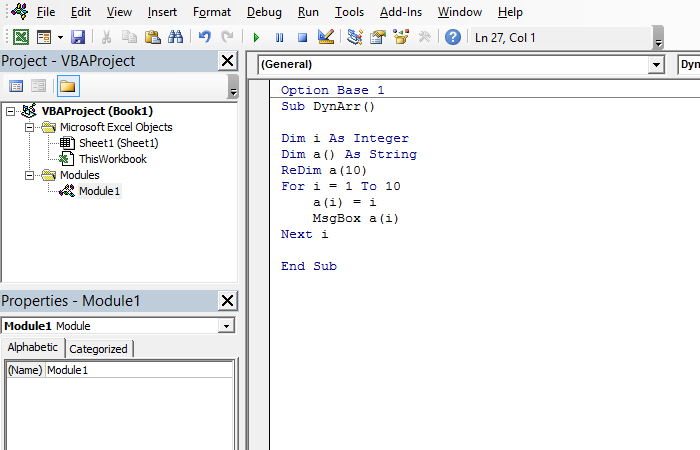# Dynamic arrays

Sometimes we need to make an array that we are not sure of how many members will have. In this case should create dynamic arrays that, unlike the usual ones, do not have a fixed length. These arrays are declared in such a way that the number of members is omitted, and how many of them will be, or for how long the number of members of array should be increased is subsequently defined with commands that will be discussed in the text that follows.

The declaration of a dynamic array is done as follows:

Dim a() As Integer

As you can see, the procedure is the same as when declaring a regular array, except that we omitted the parameter by which number of its members is assigned. Before we start using this series, we need to determine how many members will be. This is accomplished with the command:

ReDim <array name>(<array size>)

For example, if we want to declare dynamic array and further set its size to 10 members should write following code:

Dim a() As String
ReDim a(9)

Each time the ReDim command is run the array is initialized or, differently said, its content is deleted. If we wanted, for example, to add another 10 members of a string without having to delete the values of members, we would write this command using the additional Preserve parameter:

Dim a() As String
ReDim Preserve a(19)

Činjenica da indeksiranje članova niza počinje od nule može delovati zbunjujuće, pa uvek možete da naglasite da indeksi polaze od broja 1. U tom slučaju to treba da naglasite na početku modula, a zatim prilikom zadavanja veličine niza unosite pravi broj članova. Evo jednog programa koji to ilustruje:

The fact that indexing members of an array starts from zero value can be confusing, so you can always emphasize that the indexes start from the number 1. In this case, you should declare this at the beginning of the module, then enter the right number of members when setting the size of array. Here is a program that illustrates this:

Option Base 1

Sub DynArr()

Dim i As Integer
Dim a() As String
ReDim a(10)
For i = 1 To 10
a(i) = i
MsgBox a(i)
Next i

End Sub